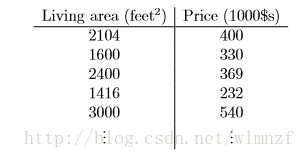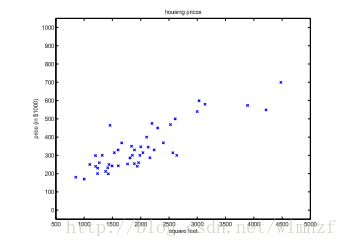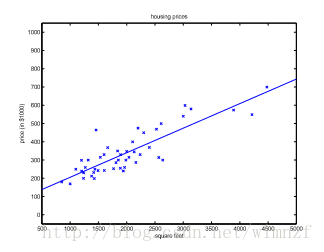#### 前言

本来对数学没什么感觉的，但是停摆了一年复习考研，于是开始对数学有些感觉了，之前看到《机器学习实战》中第五章中梯度上升法，使用了一个它所谓的十分简单的推导，一直好奇怎么个简单法，于是重新学习机器学习的相关算法，这次将主推数学推导。

#### 有监督回归算法

在机器学习中，多元线性回归模型是经常使用的模型，比如在吴恩达《斯坦福机器学习》中的例子，我们需要根据已有的房价信息预测当前房子的房价，于是我们收集到一些房价数据。再将它们画在二维坐标上，它们就以离散的点分布在平面上，如下所示我们希望能根据这些已知的点来预测我们想知道的房子的房价，因此我们需要找到一条规律，也就是一条大致经过这些点的线性模型，在数学上我们通常称之为拟合，而这个拟合的过程，我们称之为回归。假设我们建立的模型是一元一次的，将得到这样的拟合结果，于是我们可以x轴上的房屋面积，找到对应的房屋价格。

有监督的学习算法，可以理解成我们训练模型的时候每一个输入都是有标准答案的，我们通过预测值跟标准答案的比对，不断修改模型的参数才能最终实现较好地的拟合结果。

#### 最小二乘法

最小二乘法是我们经常使用的拟合算法，它通过最小化误差的平方和寻找数据的最佳函数匹配。

以我们《机器学习实战》第五章作例子，我们假设的模型为z，于是函数即可设为

$$\begin{equation} z=w0+w_1x_1+w2x2+w3x3+….+w_nx_n\\=w_0x_0+w_1x_1+w2x2+w3x3+….+w_nx_n (x0=1) \tag{1} \end{equation}$$

这种写法也可以表示为向量的写法:

$$z=w^Tx= \begin{bmatrix} w_0&w_1&…&w_n \end{bmatrix} \begin{bmatrix} x_0\\ x_1\\ …\\ x_n\\ \end{bmatrix} \tag{2}$$

同样的道理，我们也可以这样子表示

$$z=x^Tw= \begin{bmatrix} x_0&x_1&…&x_n \end{bmatrix} \begin{bmatrix} w_0\\ w_1\\ …\\ w_n\\ \end{bmatrix} \tag{3}$$

刚才我们也提到了，最小二乘法拟合的原理是最小化误差的平方和，我们将这个平方和称为损失函数，跟我们平时常用的方差类似，当这个损失函数越小，我们的模型就越能跟离散的点匹配起来:

$$f(w)=\frac{1}{2} \sum_{i=1}^m(z_w( x_i) -y_j )^2 \tag{4}$$

因为梯度上升算法是用来计算函数的最大值的，而梯度下降算法则是计算函数最小值的。而我们的损失函数自然是越小越好，我们需要求得一个系数来使得f(w)最小，可是使用梯度上升法是用于求最大值的，因此为了用上梯度上升算法，我们最终应该在f(w)前加上负号。假设:

$$J(w)=-f(w) \tag{5}$$

$$\begin{equation} X= \begin{bmatrix} x_{11}&x_{12}&…&x_{1n}\\ x_{21}&x_{22}&…&x_{2n}\\ …&…&…&…\\ x_{m1}&x_{m2}&…&x_{mn}\ \end{bmatrix} = \begin{bmatrix} x_1^{T}\\ x_2 ^{T}\\ …\\ x_m\\ \end{bmatrix} \tag{6} \end{equation}$$

$$Xw= \begin{bmatrix} x_1^T\\ x_2^T\\ …\\ x_m^T\\ \end{bmatrix} w= \begin{bmatrix} x_1^Tw\\ x_2^Tw\\ …\\ x_m^Tw\\ \end{bmatrix}= \begin{bmatrix} z_w(x_1)\\ z_w(x_2)\\ …\\ z_w(x_m)\\ \end{bmatrix} \tag{7}$$

$$Xw-\overrightarrow{y}= \begin{bmatrix} x_1^Tw\\ x_2^Tw\\ …\\ x_m^Tw\\ \end{bmatrix}- \begin{bmatrix} y_1\\ y_2\\ …\\ y_m\\ \end{bmatrix}= \begin{bmatrix} z_w(x_1)-y_1\\ z_w(x_2)-y_2\\ …\\ z_w(x_m)-y_m \end{bmatrix} \tag{8}$$

$$\because z^Tz=\sum_i^nz_i^2 \tag{9}$$

$$\therefore \frac{1}{2}(Xw-\overrightarrow{y})^T(Xw-\overrightarrow{y})= \frac{1}{2}\sum_{i=1}^n(z_w(x_i)-y_i)^2=f(w) \tag{10}$$

$$\begin{equation} \begin{split} &\nabla_wf(w)=\nabla_w\frac{1}{2}(Xw-\overrightarrow{y})^T(Xw-\overrightarrow{y})\\ &=\frac{1}{2}\nabla_w(w^TX^TXw-w^TX^T\overrightarrow{y}-\overrightarrow{y}^TXw+\overrightarrow{y}^T\overrightarrow{y})\\ &=\frac{1}{2}\nabla_wtr(w^TX^TXw-w^TX^T\overrightarrow{y}-\overrightarrow{y}^TXw+\overrightarrow{y}^T\overrightarrow{y}) \\ &=\frac{1}{2}\nabla_w(trw^TX^TXw-2tr\overrightarrow{y}^TXw) \\ &=\frac{1}{2}(X^TXw+X^TXw-2X^T\overrightarrow{y})\\ &=X^TXw-X^T\overrightarrow{y}=X^T(Xw-\overrightarrow{y})\\\\ &=>J(w)=-f(w)=X^T(\overrightarrow{y}-Xw) \end{split} \end{equation} \tag{11}$$

dataMatrix=X;
weights=w;
labelMat=y;

#### 参考

##### 来源

http://csuncle.com/2017/06/13/《机器学习实战》-chapter5梯度上升算法-数学推导/Welcome！
I'm happy it's useful to you!
Show comments from Gitment